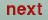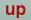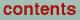Next: Density of states plot Up: Plot programs Previous: Hartree potential plot

## Band structure plot

The program gnubnd.run generates plots of the band structure along the symmetry lines defined in the SYML category of the CTRL file. The program reads the CTRL file, the BNDS file and, if FATBAND=T in the category options in the CTRL file, the file EIGN. The latter two are created by lmbnd.run. It creates the plot data files BNDS.DAT, FERMI.DAT and the command file BNDS.GNU. For an orbital projected bands plot BNDS2-4.DAT are created in addition.

After the selection of the output device one is prompted for a 40-character title string for the plot:

` enter title:`
followed by the definition of the energy unit:
` energies in Ryd.(f) or eV(t)? (default f)`
Entering t (logical true) selects electron volts, whereas f (logical false) or / selects Rydberg. The next prompt permits you to shift the origin of the energy scale to the Fermi energy:
`  energies relative to EF (t)? (default f)`
Depending on the choice of symmetry lines and energy range it may be advantageous to plot the band structure in portrait mode instead of the default landscape mode:
`  landscape plot (t) ? (default t)`
To achieve this enter f (logical false) after this prompt. If subsequent points of the same band should not be connected by a line, enter f (logical false) at the next prompt:
`  energies connected by lines (t)? (default t)`
The decision whether orbital projected bands are to be plotted or not is the next issue:
`  plot orbital character(t)? (default f)`
Enter t (logical true) to plot orbital projected bands, whereas f (logical false) or / result in an ordinary band plot. If the fat bands option is not used you may skip to the modification of the energy range below. If the fatband plot option is chosen, the prompted is:
```Change coordinate system? Enter Euler angles:
alpha, beta, gamma(units of Pi).
If nochange: enter "/"```
If the internal coordinate system should be changed, enter the Euler angles. Enter / to retain the current orientation. Now the atoms whose orbitals should be displayed fat should be given:
```no coordinate transformation!
Enter orbital character plotted as "fatbands"
First, enter name(s) of atom(s) format(20a4)
followed by <enter> and "/" on next line```
Enter the names of the atoms as specified in the CLASS category of the CTRL file separated by blanks. Enter `/' on the next line. If there are several atoms of this class, can be selected:
```RB1
Following atom(s) selected RB1
There are  6  atoms of type RB1
Specify which one(s) to select, e.g. 1 3 7 for
the first, third, and seventh, followed by "/"```
Enter a list of integers followed by `/'. Next the orbitals to be included in the fatband plot should be given:
```For each atom specify orbital number from list:
NB! Orbitals are in the new coordinate system!
s  y  z  x  xy  yz  3z^2-1  xz  x^2-y^2
1  2  3  4   5   6     7     8     9
y(3x^2-y^2) xyz y(5z^2-1) z(5z^2-3)
10      11   12        13
x(5z^2-1) z(x^2-y^2) x(3y^2-x^2)
14         15          16
RB1 number 1 enter number(s) followed by "/":```
Again, enter a list of integers followed by `/'. This step is repeated for all selected atoms of all selected classes. Now all fat band specific options have been set. The input files are read in and some information about them is displayed:
``` Bands= 44 Fermi Energy= -.1426
Lattice const.=29.589 Spins=1
nq1=   35
nq1=   40
nq1=   20
nq1=   30
nq1=   25
nq1=   45
nq1=    0
ebot=-.3864 etop=.3131 eferm=-.14258
nq=  195   nline=    5
default emin and emax =  -1.0 1.0```
If band structure plot should be limited to a fraction of the calculated energy range the new boundaries may be entered after this prompt:
` enter emin, emax`
Entering / instead of two scalars selects the whole range shown above. Now the plotfile or the plot window should be generated.

Important notice: Gnuplot is currently not capable of handling more than one font per plot. Therefore it is impossible to mix greek and roman letters and e.g. the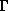point is represented by G. If the output is postscript, however, it is possible to edit the file bnds.ps and replace the sequence
( G ) Cshow
by
/Symbol findfont 180 scalefont setfont
( G ) Cshow
/Times-Roman findfont 180 scalefont setfont
.

One can move the label of the y-axis closer to the plot, simply by changing the line
currentpoint gsave translate 90 rotate 0 0 moveto ( Energy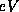) Cshow
to
currentpoint gsave translate 90 rotate 0 -700 moveto ( Energy) Cshow.Next: Density of states plot Up: Plot programs Previous: Hartree potential plot

O. Jepsen
Thu Oct 12 14:48:45 MESZ 2000